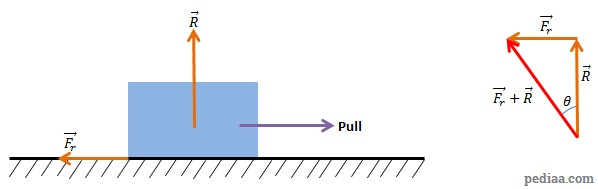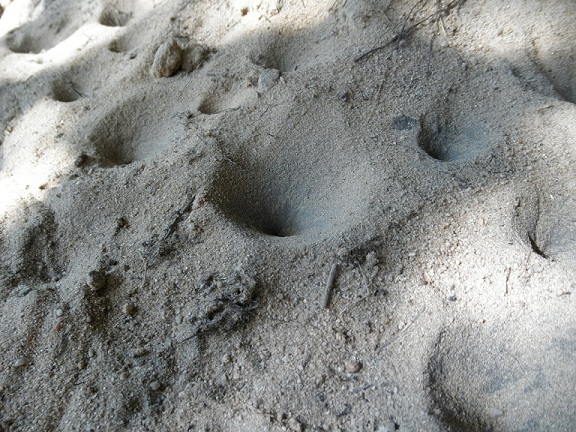# Difference Between Angle of Friction and Angle of Repose

## Main Difference –Angle of Friction vs. Angle of Repose

Angle of friction and angle of repose are two angles that come up in mechanical engineering. The angle of repose also has a use in soil mechanics. The main difference between angle of friction and angle of repose is that the angle of friction is defined as the angle between the normal reaction force and the resultant force of normal reaction force and friction when an object just begins to move, whereas the angle of repose is defined as the minimum angle of an inclined plane which causes an object to slide down the plane. We will see later that these two definitions are equivalent.

## What is Angle of Friction

Angle of friction is defined as the angle made between the normal reaction force and the resultant force of normal reaction force and friction. Let us first explore this definition and attempt to express the angle of friction in terms of a formula.Defining angle of friction

The diagram above shows a block being pulled against a rough surface. If the force is small, the static friction ($F_r$) between the surfaces would prevent the block from moving. If you slowly increase the pull,$F_r$ would also increase, balancing the horizontal forces and still preventing the block from moving. However, at one point, the block would just begin to move. At this point, the static friction has reached the upper limit, and we can describe this maximum friction as$F_r=\mu R$ where$\mu$ is the coefficient of friction and$R$ is the normal reaction force.

The vector diagram on the right shows the sum of$F_r$ and$R$. From the definition we had above,$\theta$ is the angle of friction. Using trigonometry, we can express this angle$\theta$ as$\mathrm{tan\:}\theta=\frac{F_r}{R}=\frac{\mu R}{R}=\mu$. i.e., the angle of friction between two surfaces is equal to the inverse tangent of the coefficient of friction between those two surfaces.$\theta=\mathrm{tan^{-1}}\:\mu$

## What is Angle of Repose

The angle of repose is the maximum angle that a surface can be tilted from the horizontal, such that an object on it is just able to stay on the surface without it sliding down. Again, let us first look at the diagram of the situation.

When the object is just about to move, the size of friction is given by$F_r=\mu R$. The object is also in equilibrium (the object is about to move, but it is not moving yet!) so taking the forces acting along the plane, we can say,$F_r=mg\:\mathrm{sin\:}\phi=\mu R$.

Resolving forces perpendicular to the plane, we have,$mg\:\mathrm{cos\:}\phi= R$

Now, we take the ratio of these expressions:$\frac{mg\:\mathrm{sin\:}\phi}{mg\:\mathrm{cos\:}\phi}=\frac{\mu R}{R}$.

Using the trigonometric identity$\frac{\mathrm{sin\:}\phi}{\mathrm{cos\:}\phi}=\mathrm{tan\:}\phi$, and cancelling out common factors. we get$\mathrm{tan\:}\phi =\mu$. So, we get:$\phi =\mathrm{tan^{-1}\:}\mu$

For angle of friction$\theta$, we had$\theta =\mathrm{tan^{-1}\:}\mu$ and we see that this is the same expression that we get for angle of repose as well. Therefore, angle of friction is equal to angle of repose.

In soil mechanics, the angle of repose refers to the shallowest angle of a pile of soil that causes soil particles begin to fall down. Antlions make use of this angle when they build their traps (see below):Antlions make these sand traps to catch ants and other small insects. The sides of these traps are at the angle of repose. When ants wander into the pit, the disturbance causes the sand to begin rolling down, making it difficult for the ant to escape. Then the antlion, which had been hiding at the bottom of the trap, comes out and catches the ant.

## Difference Between Angle of Friction and Angle of Repose

Essentially, the angle of repose and angle of friction are the same, given by the inverse tangent of the coefficient of friction between two surfaces. Their difference lies in the way they are described.

### Description

Angle of Friction is the angle made between the normal reaction force and the resultant force between friction and normal reaction force, when an object just begins to move along the surface.

Angle of Repose is the shallowest angle of an incline where an object on it will begin to slide down.

### Where the description applies

The definition for angle of friction can be applied to any type of surface.

The definition for angle of repose can only be used when there is an inclined surface.

References

Bansal, R. K. (2002). A Textbook of Engineering Mechanics. New Delhi: Laxmi Publications.

Image Courtesy

“sand pit traps of antlions 2” by Michal Maňas (Own work) [CC BY-SA 2.0], via flickr